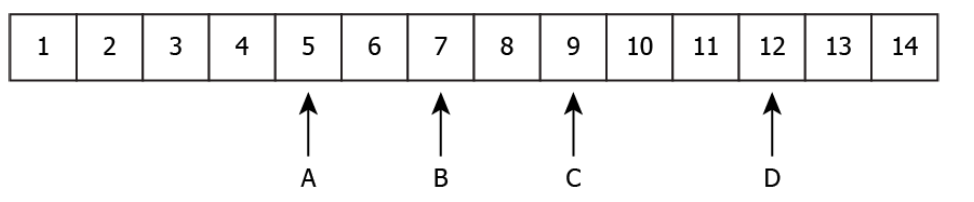# CBSE Class 10 Science MCQ Chapter 2 Acids Bases and Salt

Are you looking for multiple choice questions of CBSE Class 10 Science Chapter 2 Acids Bases and Salt? Then, you have landed on the right page. Here, we have compiled the questions of varying difficulty levels to help students in their exam preparation. They can easily download the CBSE Class 10 Science MCQ Chapter 2 Acids Bases and Salt PDF from the link below.

These questions are prepared as per the CBSE Class 10 Science Syllabus and cover Chapter 2 Acids Bases and Salt important concepts. Practising these MCQs will boost the confidence level of students so that they can attempt the exam boldly. Students can solve the CBSE Class 10 Science Sample Papers based on the latest paper design for more practice.

## CBSE Class 10 Science MCQ Chapter 2 Acids Bases and Salt with Answers

Q1) Which Acid is present in Tomato ?

(A) Citric Acid

(B) Oxalic Acid

(C) Lactic Acid

(D) HCl

Q2) Which Acid is a strong Acid in the following:

(A) HCl pH 1

(B) CH3COOH pH 5

(C) Lemon juice pH 2.2

(D) Pure Milk pH 6

Q3) Na2CO3.10H2O is known as-

(A) Baking Soda

(B) Baking Powder

(C) Washing Soda

(D) Bleaching Powder

Q4) pH value less than 7 indicates that the solution is –

(A) Acidic

(B) Basic

(C) Neutral

(D) No effect

Q5) Which salt is Neutral salt?

(A) NH4Cl

(B) CH3COONH4

(C) CH3COONa

(D)Na2CO3

Q6) Lactic Acid is present in-

(A) Orange

(B) Tea

(C) Curd

(D) Vinegar

Q7) Farmers neutralize the effect of Acidity on soil by adding

(A) Slaked Lime

(B) Gypsum

(C) Caustic Soda

(D) Baking Soda

Q8) Which of the following are present in a dilute Aqueous solution of Hydrochloric Acid?

(A) H3O + Cl

(B) H3O + OH

(C) Cl + OH

(D) Unionised HCl

Q9) CuSO4.5H2O In this Compound the water molecule is called –

(A) Pure Water

(B) Water of Crystallisation

(C) Soda Water

(D) None of these

Q10) Which of the following salts does not contain water of crystallization?

(A) Blue vitriol

(B) Baking soda

(C) Washing soda

(D) Gypsum

Q11) An aqueous solution turns the red litmus solution blue. Excess addition of which of the following solutions would reverse the change?

(A) Baking powder

(B) Lime

(C) Ammonium hydroxide solution

(D) Hydrochloric acid

Q12) In which pH range does our body work to survive in the atmosphere?

(A) 5.5 to 8.5

(B) 7.0 to 7.8

(C) 2.3 to 7.0

(D) 7.5 to 12.5

Q13) What is the chemical formula of POP (Plaster of Paris)?

(A) CaSO4.2H2O

(B) CaSO4.3H2O

(C) CaSO4.1/2H2O

(D) CaCO3.1/2H2

Q14) What happens when a solution of an acid is mixed with a solution of a base in a test tube?

(i) The temperature of the solution increases

(ii) The temperature of the solution decreases

(iii) The temperature of the solution remains the same

(iv) Salt formation takes place

(A) (i) only

(B) (i) and (iii)

(C) (ii) and (iii)

(D) (i) and (iv)

Q15) Which salt is Acidic in nature?

(A) NH4Cl

(B) CH3COONH4

(C) NaCl

(D)Na2CO3

Q16) When a base reacts with a metal, it forms a salt and hydrogen gas is released. By what method can the presence of hydrogen be detected?

(A) by water

(B) by litmus paper

(C) by methyl orange

(D) by a burning candle

Q17) The image shows the pH values of four solutions on a pH scale.Which solutions are alkaline in nature?

(A) A and B

(B) B and C

(C) C and D

(D) A and D